# Samacheer Kalvi Books: Tamilnadu State Board Text Books Solutions

## Samacheer Kalvi 10th Maths Chapter 2 Numbers and Sequences Notes PDF Download: Tamil Nadu STD 10th Maths Chapter 2 Numbers and Sequences NotesSamacheer Kalvi 10th Maths Chapter 2 Numbers and Sequences Notes PDF Download: Tamil Nadu STD 10th Maths Chapter 2 Numbers and Sequences Notes

## Samacheer Kalvi 10th Maths Chapter 2 Numbers and Sequences Notes PDF Download

We bring to you specially curated Samacheer Kalvi 10th Maths Chapter 2 Numbers and Sequences Notes PDF which have been prepared by our subject experts after carefully following the trend of the exam in the last few years. The notes will not only serve for revision purposes, but also will have several cuts and easy methods to go about a difficult problem.

 Board Tamilnadu Board Study Material Notes Class Samacheer Kalvi 10th Maths Subject 10th Maths Chapter Chapter 2 Numbers and Sequences Format PDF Provider Samacheer Kalvi Books

## How to Download Samacheer Kalvi 10th Maths Chapter 2 Numbers and Sequences Notes PDFs?

2. Click on the Samacheer Kalvi 10th Maths Notes PDF.
3. Look for your preferred subject.
4. Now download the Samacheer Kalvi 10th Maths Chapter 2 Numbers and Sequences notes PDF.

## Tamilnadu Samacheer Kalvi 10th Maths Solutions Chapter 2 Numbers and Sequences Ex 2.1

Exercise 2.1 Class 10 Maths Samacheer Question 1.
Find all positive integers which when divided by 3 leaves remainder 2.
The positive integers when divided by 3 leaves remainder 2.
By Euclid’s division lemma a = bq + r, 0 ≤ r < b.
Here a = 3q + 2, where 0 ≤ q < 3, a leaves remainder 2 when divided by 3.
∴ 2, 5, 8, 11 ……………..

10th Maths Exercise 2.1 Samacheer Kalvi Question 2.
A man has 532 flower pots. He wants to arrange them in rows such that each row contains 21 flower pots. Find the number of completed rows and how many flower pots are left over?
Here a = 532, b = 21
Using Euclid’s division algorithm
a = bq + r
532 = 21 × 25 + 7
Number of completed rows = 21
Number of flower pots left over = 7

Ex 2.1 Class 10 Samacheer Question 3.
Prove that the product of two consecutive positive integers is divisible by 2.
Solution:
Let n – 1 and n be two consecutive positive integers. Then their product is (n – 1)n.
(n – 1)(n) = n2 – n.
We know that any positive integer is of the form 2q or 2q + 1 for some integer q. So, following cases arise.
Case I. When n = 2q.
In this case, we have
n2 – n = (2q)2 – 2q = 4q2 – 2q = 2q(2q – 1)
⇒ n2 – n = 2r, where r = q(2q – 1)
⇒ n2 – n is divisible by 2.

Case II. When n = 2q + 1
In this case, we have
n2 – n = (2q + 1)2 – (2q + 1)
= (2q + 1)(2q + 1 – 1) = 2q(2q + 1)
⇒ n2 – n = 2r, where r = q (2q + 1).
⇒ n2 – n is divisible by 2.
Hence, n2 – n is divisible by 2 for every positive integer n.
Hence it is Proved

10th Maths Exercise 2.1 Question 4.
When the positive integers a, b and c are divided by 13, the respective remainders are 9,7 and 10. Show that a + b + c is divisible by 13.
Let the positive integer be a, b, and c
We know that by Euclid’s division lemma
a = bq + r
a = 13q + 9 ….(1)
b = 13q + 7 ….(2)
c = 13q + 10 ….(3)
a + b + c = 13q + 9 + 13q + 7 + 13q + 10
= 39q + 26
a + b + c = 13 (3q + 2)
This expansion will be divisible by 13
∴ a + b + c is divisible by 13

10th Maths 2.1 Exercise Question 5.
Prove that square of any integer leaves the remainder either 0 or 1 when divided by 4.
Solution:
Let x be any integer.
The square of x is x2.
Let x be an even integer.
x = 2q + 0
then x2 = 4q2 + 0
When x be an odd integer
When x = 2k + 1 for some interger k.
x2 = (2k + 1 )2
= 4k2 + 4k + 1
= 4k (k + 1) + 1
= 4q + 1
where q = k(k + 1) is some integer.
Hence it is proved.

10th Maths Exercise 2.1 In Tamil Question 6.
Use Euclid’s Division Algorithm to find the Highest Common Factor (H.C.F) of
(i) 340 and 412
To find the HCF of 340 and 412 using Euclid’s division algorithm. We get
412 = 340 × 1 + 72
The remainder 72 ≠ 0
Again applying Euclid’s division algorithm to the division of 340
340 = 72 × 4 + 52
The remainder 52 ≠ 0
Again applying Euclid’s division algorithm to the division 72 and remainder 52 we get
72 = 52 × 1 + 20
The remainder 20 ≠ 0
Again applying Euclid’s division algorithm
52 = 20 × 2 + 12
The remainder 12 ≠ 0
Again applying Euclid’s division algorithm
20 = 12 × 1 + 8
The remainder 8 ≠ 0
Again applying Euclid’s division algorithm
12 = 8 × 1 + 4
The remainder 4 ≠ 0
Again applying Euclid’s division algorithm
8 = 4 × 2 + 0
The remainder is zero
∴ HCF of 340 and 412 is 4

(ii) 867 and 255
To find the HCF of 867 and 255 using
Euclid’s division algorithm. We get
867 = 255 × 3 + 102
The remainder 102 ≠ 0
Using Euclid’s division algorithm
255 = 102 × 2 + 51
The remainder 51 ≠ 0
Again using Euclid’s division algorithm
102 = 51 × 2 + 0
The remainder is zero
∴ HCF = 51
∴ HCF of 867 and 255 is 51

(iii) 10224 and 9648
Find the HCF of 10224 and 9648 using Euclid’s division algorithm. We get
10224 = 9648 × 1 + 576
The remainder 576 ≠ 0
Again using Euclid’s division algorithm
9648 = 576 × 16 + 432
The remainder 432 ≠ 0
Using Euclid’s division algorithm
576 = 432 × 1 + 144
The remainder 144 ≠ 0
Again using Euclid’s division algorithm
432 = 144 × 3 + 0
The remainder is 0
∴ HCF = 144
The HCF of 10224 and 9648 is 144

(iv) 84,90 and 120
Find the HCF of 84, 90 and 120 using Euclid’s division algorithm
90 = 84 × 1 + 6
The remainder 6 ≠ 0
Using Euclid’s division algorithm
4 = 14 × 6 + 0
The remainder is 0
∴ HCF = 6
The HCF of 84 and 90 is 6
Find the HCF of 6 and 120
120 = 6 × 20 + 0
The remainder is 0
∴ HCF of 120 and 6 is 6
∴ HCF of 84, 90 and 120 is 6

Numbers And Sequences 10th Class Question 7.
Find the largest number which divides 1230 and 1926 leaving remainder 12 in each case.
Solution:
The required number is the H.C.F. of the numbers.
1230 – 12 = 1218,
1926 – 12 = 1914
First we find the H.C.F. of 1218 & 1914 by Euclid’s division algorithm.
1914 = 1218 × 1 + 696
The remainder 696 ≠ 0.
Again using Euclid’s algorithm
1218 = 696 × 1 + 522
The remainder 522 ≠ 0.
Again using Euclid’s algorithm.
696 = 522 × 1 + 174
The remainder 174 ≠ 0.
Again by Euclid’s algorithm
522 = 174 × 3 + 0
The remainder is zero.
∴ The H.C.F. of 1218 and 1914 is 174.
∴ The required number is 174.

10th Maths Ex 2.1 Question 8.
If d is the Highest Common Factor of 32 and 60, find x and y satisfying d = 32x + 60y.
Find the HCF of 32 and 60
60 = 32 × 1 + 28 ….(1)
The remainder 28 ≠ 0
By applying Euclid’s division lemma
32 = 28 × 1 + 4 ….(2)
The remainder 4 ≠ 0
Again by applying Euclid’s division lemma
28 = 4 × 7 + 0….(3)
The remainder is 0
HCF of 32 and 60 is 4
From (2) we get
32 = 28 × 1 + 4
4 = 32 – 28
= 32 – (60 – 32)
4 = 32 – 60 + 32
4 = 32 × 2 -60
4 = 32 x 2 + (-1) 60
When compare with d = 32x + 60 y
x = 2 and y = -1
The value of x = 2 and y = -1

10th Samacheer Maths Exercise 2.1 Question 9.
A positive integer when divided by 88 gives the remainder 61. What will be the remainder when the same number is divided by 11?
Solution:
Let a (+ve) integer be x.
x = 88 × y + 61
61 = 11 × 5 + 6 (∵ 88 is multiple of 11)
∴ 6 is the remainder. (When the number is divided by 88 giving the remainder 61 and when divided by 11 giving the remainder 6).

10th Maths 2.1 Question 10.
Prove that two consecutive positive integers are always coprime.

1. Let the consecutive positive integers be x and x + 1.
2. The two number are co – prime both the numbers are divided by 1.
3. If the two terms are x and x + 1 one is odd and the other one is even.
4. HCF of two consecutive number is always 1.
5. Two consecutive positive integer are always coprime.

Fundamental Theorem of Arithmetic
Every composite number can be written uniquely as the product of power of prime is called fundamental theorem of Arithmetic.

## Tamilnadu Samacheer Kalvi 10th Maths Solutions Chapter 2 Numbers and Sequences Ex 2.2

10th Maths Exercise 2.2 Samacheer Kalvi Question 1.
For what values of natural number n, 4n can end with the digit 6?
Solution:
4n = (2 × 2)n = 2n × 2n
2 is a factor of 4n.
So, 4n is always even and end with 4 and 6.
When n is an even number say 2, 4, 6, 8 then 4n can end with the digit 6.
Example:
42 = 16
43 = 64
44 = 256
45 = 1,024
46 = 4,096
47 = 16,384
48 = 65, 536
49 = 262,144

Ex 2.2 Class 10 Samacheer Question 2.
If m, n are natural numbers, for what values of m, does 2n × 5m ends in 5?
2n is always even for any values of n.
[Example. 22 = 4, 23 = 8, 24 = 16 etc]

5m is always odd and it ends with 5.
[Example. 52 = 25, 53 = 125, 54 = 625 etc]
But 2n × 5m is always even and end in 0.
[Example. 23 × 53 = 8 × 125 = 1000
22 × 52 = 4 × 25 = 100]
∴ 2n × 5m cannot end with the digit 5 for any values of m.

Exercise 2.2 Class 10 Maths Samacheer Question 3.
Find the H.C.F. of 252525 and 363636.
Solution:
To find the H.C.F. of 252525 and 363636
Using Euclid’s Division algorithm
363636 = 252525 × 1 + 111111
The remainder 111111 ≠ 0.
∴ Again by division algorithm
252525 = 111111 × 2 + 30303
The remainder 30303 ≠ 0.
∴ Again by division algorithm.
111111 = 30303 × 3 + 20202
The remainder 20202 ≠ 0.
∴ Again by division algorithm
30303 = 20202 × 1 + 10101
The remainder 10101 ≠ 0.
∴ Again using division algorithm
20202 = 10101 × 2 + 0
The remainder is 0.
∴ 10101 is the H.C.F. of 363636 and 252525.

10th Maths Exercise 2.2 Question 4.
If 13824 = 2a × 3b then find a and b.
Solution:
If 13824 = 2a × 3b
Using the prime factorisation tree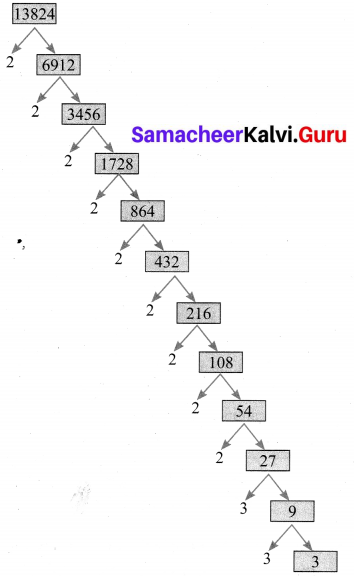13824 = 2 × 2 × 2 × 2 × 2 × 2 × 2 × 2 × 2 × 3 × 3 × 3
= 29 × 33 = 2a × 3b
∴ a = 9, b = 3.

10th Maths Exercise 2.2 In Tamil Question 5.
If p1x1 × p2x2 × p3x3 × p4x4 = 113400 where p1, p2, p3, p4 are primes in ascending order and x1, x2, x3, x4 are integers, find the value of P1, P2, P3, P4 and x1, x2, x3, x4.
Solution:
If p1x1 × p2x2 × p3x3 × p4x4 = 113400
p1, p2, p3, P4 are primes in ascending order, x1, x2, x3, x4 are integers.
using Prime factorisation tree.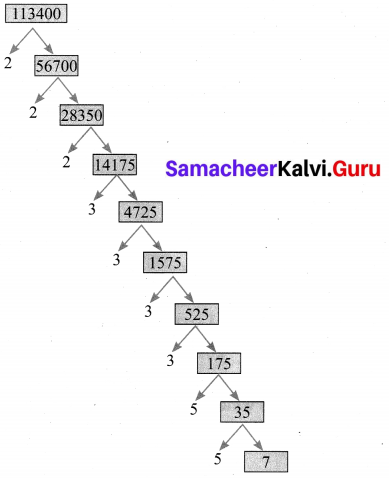113400 = 2 × 2 × 2 × 3 × 3 × 3 × 3 × 5 × 5 × 7
= 23 × 34 × 52 × 7
= p1x1 × p2x2 × p3x3 × p4x4
∴ p1= 2, p2 = 3, p3 = 5, p4 = 7, x1 = 3, x2 = 4, x3 = 2, x4 = 1.

Question 6.
Find the L.C.M. and H.C.F. of 408 and 170 by applying the fundamental theorem of arithmetic.
Solution:
408 and 170.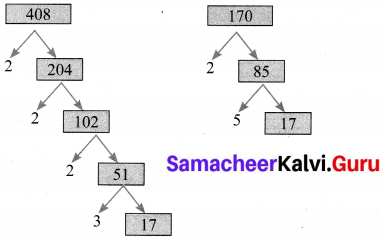408 = 23 × 31 × 171
170 = 21 × 51 × 171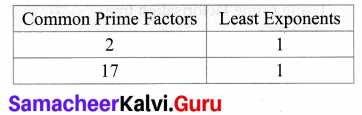∴ H.C.F. = 21 × 171 = 34.
To find L.C.M, we list all prime factors of 408 and 170, and their greatest exponents as follows.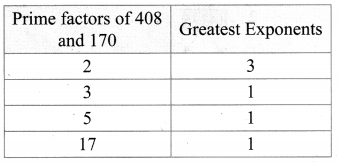∴ L.C.M. = 23 × 31 × 51 × 171
= 2040.

10th Maths 2.2 Exercise Question 7.
Find the greatest number consisting of 6 digits which is exactly divisible by 24, 15, 36?
Solution:
To find L.C.M of 24, 15, 36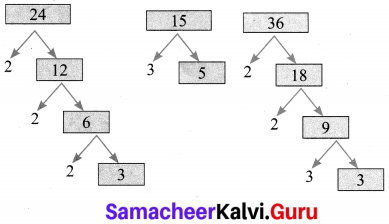24 = 23 × 3
15 = 3 × 5
36 = 22 × 32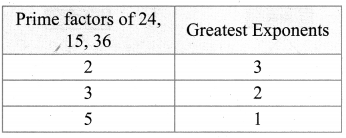∴ L.C.M = 23 × 32 × 51
= 8 × 9 × 5
= 360
If a number has to be exactly divisible by 24, 15, and 36, then it has to be divisible by 360. Greatest 6 digit number is 999999.
Common multiplies of 24, 15, 36 with 6 digits are 103680, 116640, 115520, …933120, 999720 with six digits.
∴ The greatest number consisting 6 digits which is exactly divisible by 24, 15, 36 is 999720.

10th Maths Ex 2.2 Question 8.
What is the smallest number that when divided by three numbers such as 35, 56 and 91 leaves remainder 7 in each case?
Find the L.C.M of 35, 56, and 91
35 – 5 × 7 56
56 = 2 × 2 × 2 × 7
91 = 7 × 13
L.C.M = 23 × 5 × 7 × 13
= 3640
Since it leaves remainder 7
The required number = 3640 + 7
= 3647
The smallest number is = 3647

10th Maths 2.2 Question 9.
Find the least number that is divisible by the first ten natural numbers.
Solution:
The least number that is divisible by the first ten natural numbers is 2520.
Hint:
1,2, 3,4, 5, 6, 7, 8,9,10
The least multiple of 2 & 4 is 8
The least multiple of 3 is 9
The least multiple of 7 is 7
The least multiple of 5 is 5
∴ 5 × 7 × 9 × 8 = 2520.
L.C.M is 8 × 9 × 7 × 5
= 40 × 63
= 2520

## Tamilnadu Samacheer Kalvi 10th Maths Solutions Chapter 2 Numbers and Sequences Ex 2.3

10th Samacheer Maths Exercise 2.3 Solutions Question 1.
Find the least positive value of x such that
(i) 71 ≡ x (mod 8)
(ii) 78 + x ≡ 3 (mod 5)
(iii) 89 ≡ (x + 3) (mod 4)
(iv) 96 = x7 (mod 5)
(v) 5x ≡ 4 (mod 6)
Solution:
To find the least value of x such that
(i) 71 ≡ x (mod 8)
71 ≡ 7 (mod 8)
∴ x = 7.[ ∵ 71 – 7 = 64 which is divisible by 8]

(ii) 78 + x ≡ 3 (mod 5)
⇒ 78 + x – 3 = 5n for some integer n.
75 + x = 5 n
75 + x is a multiple of 5.
75 + 5 = 80. 80 is a multiple of 5.
Therefore, the least value of x must be 5.

(iii) 89 ≡ (x + 3) (mod 4)
89 – (x + 3) = 4n for some integer n.
86 – x = 4 n
86 – x is a multiple of 4.
∴ The least value of x must be 2 then
86 – 2 = 84.
84 is a multiple of 4.
∴ x value must be 2.

(iv) 96 ≡ x7 (mod 5)
96 – x7 = 5n for some integer n.
672x7 = 5n
672 – x = 35n.
672 – x is a multiple of 35.
∴ The least value of x must be 7 i.e. 665 is a multiple of 35.

(v) 5x ≡ 4 (mod 6)
5x – 4 = 6M for some integer n.
5x = 6n + 4
x = 6n+45
When we put 1, 6, 11, … as n values in x = 6n+45 which is divisible by 5.
When n = 1, x = 105 = 2
When n = 6, x = 36+45 = 405 = 8 and so on.
∴ The solutions are 2, 8, 14…..
∴ Least value is 2.

10th Maths Exercise 2.3 Samacheer Kalvi Question 2.
If x is congruent to 13 modulo 17 then 7x – 3 is congruent to which number modulo 17?
Given x ≡ 13 (mod 17) ……(1)
7x – 3 ≡ a (mod 17) ……..(2)
From (1) we get
x- 13 = 17 n (n may be any integer)
x – 13 is a multiple of 17
∴ The least value of x = 30
From (2) we get
7(30) – 3 ≡ a(mod 17)
210 – 3 ≡ a(mod 17)
207 ≡ a (mod 17)
207 ≡ 3(mod 17)
∴ The value of a = 3

Ex 2.3 Class 10 Samacheer Question 3.
Solve 5x ≡ 4 (mod 6)
Solution:
5x ≡ 4 (mod 6)
5x – 4 = 6M for some integer n.
5x = 6n + 4
x = 6n+45 where n = 1, 6, 11,…..
∴ x = 2, 8, 14,…

10th Maths Exercise 2.3 In Tamil Question 4.
Solve 3x – 2 ≡ 0 (mod 11)
Solution:
3x – 2 ≡ 0 (mod 11)
3x – 2 = 11 n for some integer n.
3x = 11n + 2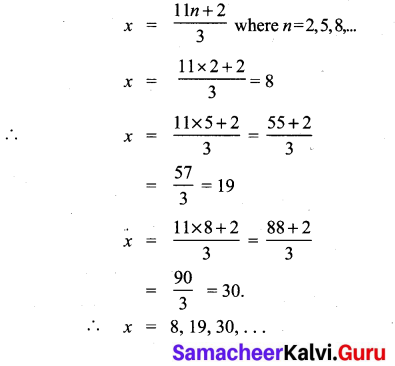Samacheer Kalvi 10th Maths Question 5.
What is the time 100 hours after 7 a.m.?
Solution:
100 ≡ x (mod 12) (∵7 comes in every 12 hrs)
100 ≡ 4 (mod 12) (∵ Least value of x is 4)
∴ The time 100 hrs after 7 O’ clock is 7 + 4 = 11 O’ clock i.e. 11 a.m

10th Maths Exercise 2.3 Question 6.
What is time 15 hours before 11 p.m.?
15 ≡ x (mod 12)
15 ≡ 3 (mod 12)
The value of x must be 3.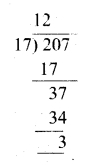The time 15 hours before 11 o’clock is (11 – 3) 8 pm

10th Maths Samacheer Kalvi Question 7.
Today is Tuesday. My uncle will come after 45 days. In which day my uncle will be coming?
Solution:
No. of days in a week = 7 days.
45 ≡ x (mod 7)
45 – x = 7n
45 – x is a multiple of 7.
∴ Value of x must be 3.
∴ Three days after Tuesday is Friday. Uncle will come on Friday.

Exercise 2.3 Class 10 Maths Samacheer Question 8.
Prove that 2n + 6 × 9n is always divisible by 7 for any positive integer n.
9 = 2 (mod 7)
9n = 2n (mod 7) and 2n = 2n (mod 7)
2n + 6 × 9n = 2n (mod 7) + 6 [2n (mod 7)]
= 2n (mod 7) + 6 × 2n (mod 7)
7 × 2n (mod 7)
It is always divisible for any positive integer n

10th Samacheer Kalvi Maths Question 9.
Find the remainder when 281 is divided by 17.
Solution:
281 ≡ x (mod 17)
240 × 240 × 241 ≡ x (mod 17)
(24)10 × (24)10 × 21 ≡ x (mod 17)
(16)10 × (16)10 × 2 ≡ x(mod 17)
(165)2 × (165)2 × 2
(165) ≡ 16 (mod 17)
(165)2 ≡ 162 (mod 17)
(165)2 ≡ 256 (mod 17)
≡ 1 (mod 17) [∵ 255 is divisible by 17]
(165)2 × (165)2 × 2 ≡ 1 × 1 × 2 (mod 17)
∴ 281 ≡ 2(mod 17)
∴ x = 2

Maths Class 10 Samacheer Kalvi Question 10.
The duration of flight travel from Chennai to London through British Airlines is approximately 11 hours. The aeroplane begins its journey on Sunday at 23:30 hours. If the time at Chennai is four and a half hours ahead to that of London’s time, then find the time in London, when will the flight lands at London Airport.
Solution:
The duration of the flight from Chennai to London is 11 hours.
Starting time at Chennai is 23.30 hrs. = 11.30 p.m.
Travelling time = 11.00 hrs. = 22.30 hrs = 10.30 a.m.
Chennai is 412 hrs ahead to London.
= 10.30 – 4.30 = 6.00
∴ At 6 a.m. on Monday the flight will reach at London Airport.

## Tamilnadu Samacheer Kalvi 10th Maths Solutions Chapter 2 Numbers and Sequences Ex 2.4

10th Maths Exercise 2.4 Samacheer Kalvi Question 1.
Find the next three terms of the following sequence.
(i) 8, 24, 72, …….
(ii) 5, 1, -3, …….
(iii) 14,29,316………..
Solution:
(i) 8, 24, 72…
In an arithmetic sequence a = 8,
d = t1 – t1 = t3 – t2
= 24 – 8 72 – 24
= 16 ≠ 48
So, it is not an arithmetic sequence. In a geometric sequence,
r = t2t1=t3t2
248=7224
⇒ 3 = 3
∴ It is a geometric sequence
∴ The nth term of a G.P is tn = arn-1
∴ t4 = 8 × 34-1
= 8 × 33
= 8 × 27
= 216

t5 = 8 × 35-1
= 8 × 34
= 8 × 81
= 648

t6 = 8 × 36-1
= 8 × 35
= 8 × 243
= 1944
The next 3 terms are 8, 24, 72, 216, 648, 1944.

(ii) 5, 1, -3, …
d = t2 – t1 = t3 – t2
⇒ 1 – 5 = -3-1
-4 = -4 ∴ It is an A.P.
tn = a+(n – 1)d
t4 = 5 + 3 × – 4
= 5 – 12
= -7
15 = a + 4d
= 5 + 4 × -4
= 5 – 16
= -11
t6 = a + 5d
= 5 + 5 × – 4
= 5 – 20
= – 15
∴ The next three terms are 5, 1, -3, -7, -11, -15.

(iii) 14,29,316,………..
Here an = Numerators are natural numbers and denominators are squares of the next numbers
14,29,316,425,536,649………….

Ex 2.4 Class 10 Samacheer Question 2.
Find the first four terms of the sequences whose nth terms are given by

(i) an = n3 -2
an = n3 – 2
a1 = 13 – 2 = 1 – 2 = -1
a2 = 23 – 2 = 8 – 2 = 6
a3 = 33 – 2 = 27 – 2 = 25
a4 = 43 – 2 = 64 – 2 = 62
The four terms are -1, 6, 25 and 62

(ii) an = (-1)n+1 n(n + 1)
an = (-1)n+1 n(n + 1)
a1 = (-1)2 (1) (2) = 1 × 1 × 2 = 2
a2 = (-1)3 (2) (3) = -1 × 2 × 3 = -6
a3 = (-1)4 (3) (4) = 1 × 3 × 4 = 12
a4 = (-1)5 (4) (5) = -1 × 4 × 5 = -20
The four terms are 2, -6, 12 and -20

(iii) an = 2n2 – 6
an = 2 n2 – 6
a1 = 2(1)2 – 6 = 2 – 6 = -4
a2 = 2(2)2 – 6 = 8 – 6 = 2
a3 = 2(3)2 – 6 = 18 – 6 = 12
a4 = 2(4)2 – 6 = 32 – 6 = 26
The four terms are -4, 2, 12, 26

10th Maths Exercise 2.4 Question 3.
Find the nth term of the following sequences
(i) 2, 5, 10, 17, ……….
(ii) 0, 12, 23,…..
(iii) 3, 8, 13, 18, ………
Solution:
(i) 2, 5, 10, 17
= 12 + 1, 22 + 1, 32 + 1, 42 + 1 ……….
∴ nth term is n2+1
(ii) 0, 12,23,………….
= 111,212,313…..
n1n
∴ nth term is n1n
(iii) 3, 8, 13, 18
a = 3
d = 5
tn = a + (n – 1)d
= 3 + (n – 1)5
= 3 + 5n – 5
= 5n – 2
∴ nth term is 5n – 2

10th Maths 2.4 Exercise Question 4.
Find the indicated terms of the sequences whose nth terms are given by
(i) an = 5nn+2 ; a6 and a13
(ii) an = -(n2 – 4); a4 and a11
Solution: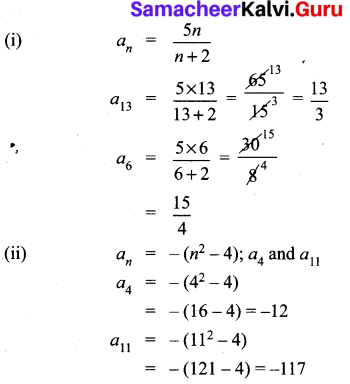10th Maths Exercise 2.4 In Tamil Question 5.
Find a8 and a15 whose nth term is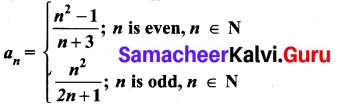Solution: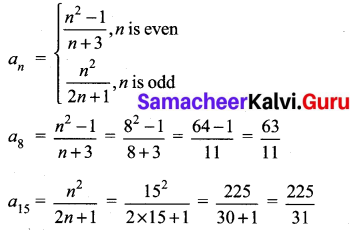Exercise 2.4 Class 10 Samacheer Kalvi Question 6.
If a1 = 1, a2 = 1 and an = 2an-1 + an-2 n > 3, n ∈ N. Then find the first six terms of the sequence.
a1 = a2 = 1
an = 2an-1 + an-2
a3 = 2a3-1 + a3-2 = 2a2 + a1
= 2(1) + 1 = 3
a4 = 2a4-1 + a4-2
= 2a3 + a2
= 2(3) + 1 = 6 + 1 = 7
a5 = 2 a5-1 + a5-2
= 2a4 + a3
= 2(7) + 3 = 17
a6 = 2a6-1 + a6-2
= 2a5 + a4
= 2(17) + 7
= 34 + 7 = 41
The sequence is 1, 1, 3, 7, 17,41, …

## Tamilnadu Samacheer Kalvi 10th Maths Solutions Chapter 2 Numbers and Sequences Ex 2.5

10th Maths Exercise 2.5 Samacheer Kalvi Question 1.
Check whether the following sequences are in A.P.
(i) a – 3, a – 5, a – 7, ………
(ii) 12,13,14,14, ………..
(iii) 9, 13, 17, 21, 25, ………
(iv) 13,0,13,23,………..
(v) 1,-1, 1,-1, 1,-1,…
Solution:
To prove it is an A.P, we have to show d = t2 – t1 = t3 – t2.
(i) a – 3, a – 5, a – 7………
t1,t2,t3
d = t2 – t1 = a – 5 – (a – 3) = a – 5 – a + 3 = -2
∴ d = -2 ∴ It is an A.P.
d = t3 – t2 = a – 7 – (a – 5) = a – 7 – a + 5 = -2

(ii) 12,13,14,14, ………..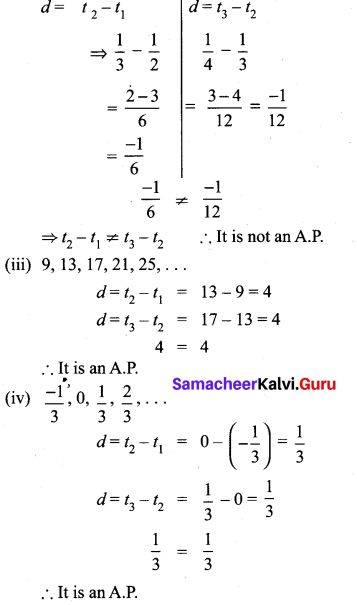(v) 1,-1, 1,-1, 1,-1,…
d = t2 – t1 = -1 -1 = -2
d = t3 – t2 = 1 – (-1) = 2
-2 ≠ 2 ∴ It is not an A.P.

Ex 2.5 Class 10 Samacheer Question 2.
First term a and common difference d are given below. Find the corresponding A.P.
(i) a = 5, d = 6
(ii) a = 7, d = 5
(iii) a = 34, d = 12
Solution:
(i) a = 5, d = 6
A.P a, a + d, a + 2d, ………
= 5, 5 + 6, 5 + 2 × 6, ………
= 5, 11, 17,…
(ii) a = 7,d = -5
A.P. = a,a + d,a + 2d,…
= 7,7 + (-5), 7 + 2(-5), ……….
= 7, 2, -3, …….,…
(iii) a = 34, d = 12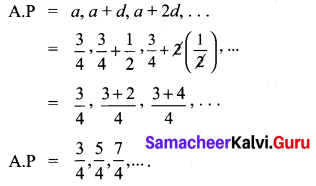Exercise 2.5 Class 10 Maths Solution Question 3.
Find the first term and common difference of the Arithmetic Progressions whose nthterms are given below

(i) tn = -3 + 2n
tn = -3 + 2 n
t1 = -3 + 2(1) = -3 + 2
= -1
t2 = -3 + 2(2) = -3 + 4
= 1
First term (a) = -1 and
Common difference
(d) = 1 – (-1) = 1 + 1 = 2

(ii) tn = 4 – 7n
tn = 4 – 7n
t1 = 4 – 7(1)
= 4 – 7 = -3
t2 = 4 – 7(2)
= 4 – 14 = -10
First term (a) = – 3 and
Common difference (d) = 10 – (-3)
= – 10 + 3
= – 7

10th Maths Exercise 2.5 Question 4.
Find the 19th term of an A.P. -11, -15, -19, ………..
Solution:
A.P = -11, -15, -19, ……..
a = -11
d = t2 – t1 =-15-(-11)
= -15 + 11
= -4
n = 19
∴ tn = a + (n – 1)d
t19 = -11 + (19 – 1)(-4)
= -11 + 18 × -4
= -11 – 72
= -83

Class 10 Maths Exercise 2.5 Solution Question 5.
Which term of an A.P. 16, 11, 6, 1,… is -54?
First term (a) = 16
Common difference (d) = 11 – 16 = -5
tn = – 54
a + (n – 1) d = -54
16 + (n – 1) (-5) = -54
54 + 21 = -54
54 + 21 = 5n
75 = 5n
n = 755 = 15
The 15th term is – 54

Samacheer Kalvi 10th Maths Exercise 2.5 Question 6.
Find the middle term(s) of an A.P. 9, 15, 21, 27, ……. ,183.
Solution:
A.P = 9, 15, 21, 27,…, 183
No. of terms in an A.P. is
a = 9, l = 183, d = 15 – 9 = 6
∴ n = 18396 + 1
= 1746 + 1
= 29 + 1 = 30
∴ No. of terms = 30. The middle must be 15th term and 16th term.
∴ t15 = a + (n – 1)d
= 9 + 14 × 6
=9 + 84
= 93
t16 = a + 15 d
= 9 + 15 × 6
= 9 + 90 = 99
∴ The middle terms are 93, 99.

10th Maths Exercise 2.5 In Tamil Question 7.
If nine times the ninth term is equal to the fifteen times fifteenth term, Show that six times twenty fourth term is zero.
tn = a + (n – 1)d
9 times 9th term = 15 times 15th term
9t9 = 15 t15
9[a + 8d] = 15[a + 14d]
9a + 72d = 15a + 210d
9a – 15a + 72 d – 210 d = 0
-6a – 138 d = 0
6a + 138 d = 0
6 [a + 23 d] = 0
6 [a + (24 – 1)d] = 0
6 t24 = 0
∴ Six times 24th terms is 0.

Ex 2.5 Class 10 Question 8.
If 3 + k, 18 – k, 5k + 1 are in A.P. then find k.
Solution:
3 + k, 18 – k, 5k + 1 are in A.P
⇒ 2b = a + c if a, b, c are in A.P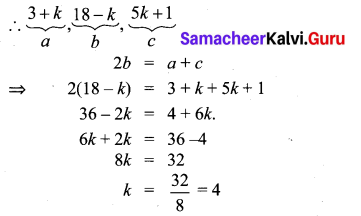10th Maths 2.5 Exercise Question 9.
Find x,y and z gave that the numbers x,
10, y, 24, z are in A.P.
x, 10, y, 24, z are in A.P
t2 – t1 = 10 – x
d = 10 – x …..(1)
t3 – t2 = y – 10
d = y – 10 ……(2)
t4 – t3 = 24 – y
d = 24 – y …..(3)
t5 – t4 = z – 24
d = z – 24 …..(4)
From (2) and (3) we get
y – 10 = 24 – y
2y = 24 + 10
2y = 34
y = 17
From (1) and (2) we get
10 – x = y – 10
– x – y = -10 -10
-x -y = -20
x + y = 20
x + 17 = 20(y = 17)
x = 20 – 17 = 3
From (1) and (4) we get
z – 24 = 10 – x
z – 24 = 10 – 3 (x = 3)
z – 24 = 7
z = 7 + 24
z = 31
The value of x = 3, y = 17 and z = 31

10th Maths 2.5 Question 10.
In a theatre, there are 20 seats in the front row and 30 rows were allotted. Each successive row contains two additional seats than its front row. How many seats are there in the last row?
Solution:
t1 = a = 20
t2 = a + 2 = 22
t3 = a + 2 + 2 = 24 ⇒ d = 2
∴ There are 30 rows.
t30 = a + 29d
= 20 + 29 × 2
= 20 + 58
= 78
∴ There will be 78 seats in the last row.

Exercise 2.5 Class 10 Question 11.
The Sum of three consecutive terms that are in A.P. is 27 and their product is 288. Find the three terms.
Let the three consecutive terms be a – d, a and a + d
By the given first condition
a – d + a + a + d = 27
3a = 27
a = 273 = 9
Again by the second condition
(a – d) (a) (a + d) = 288
a (a2 – d2) = 288
9(81 – d2) = 288 (a = 9)
81 – d2 = 2889
81 – d2 = 32
∴ d2 = 81 – 32
= 49
d = 49−−√ = ± 7
When a = 9, d = 7
a + d = 9 + 7 = 16
a = 9
a – d = 9 – 7 = 2
When a = 9, d = -7
a + d = 9 – 7 = 2
a = 9
a – d = 9 – (-7) = 9 + 7 = 16
The three terms are 2, 9, 16 (or) 16, 9, 2

Maths Exercise 2.5 Class 10 Question 12.
The ratio of 6th and 8th term of an A.P is 7:9. Find the ratio of 9th term to 13th term.
Solution:
t6t8=79
a+5da+7d = 79
9a + 45d = 7a + 49d
9 a + 45 – 7d = 7a + 49 d
9a + 45d – 7a – 49d = 0
2a – 4d = 0 ⇒ 2a = 4d
a = 2d
Substitue a = 2d in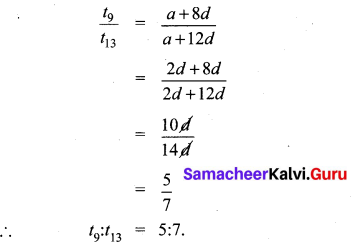Exercise 2.5 Class 10 Maths Samacheer Question 13.
In a winter season let us take the temperature of Ooty from Monday to Friday to be in A.P. The sum of temperatures from Monday to Wednesday is 0° C and the sum of the temperatures from Wednesday to Friday is 18° C. Find the temperature on each of the five days.
Solution:
Let the five days temperature be (a – d), a, a + d, a + 2d, a + 3d.
The three days sum = a – d + a + a + d = 0
⇒ 3a = 0 ⇒ a = 0. (given)
a + d + a + 2d + a + 3d = 18
3a + 6d = 18
3(0) + 6 d = 18
6d = 18
d = 186 = 3
∴ The temperature of each five days is a – d, a, a + d, a + 2d, a + 3d
0 – 3, 0, 0 + 3, 0 + 2(3), 0 + 3(3) = -3°C, 0°C, 3°C, 6°C, 9°C

2.5 Exercise Class 10 Question 14.
Priya earned ₹ 15,000 in the first month. Thereafter her salary increased by ₹1500 per year. Her expenses are ₹13,000 during the first year and the expenses increases by ₹900 per year. How long will it take for her to save ₹20,000 per month.
Solution: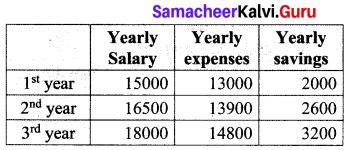We find that the yearly savings is in A.P with a1 = 2000 and d = 600.
We are required to find how many years are required to save 20,000 a year …………..
an = 20,000
an = a + (n – 1)d
20000 = 2000 + (n – 1)600
(n – 1)600 = 18000
n – 1 = 18000600 = 30
n = 31 years

## Tamilnadu Samacheer Kalvi 10th Maths Solutions Chapter 2 Numbers and Sequences Ex 2.6

10th Maths Exercise 2.6 Samacheer Kalvi Question 1.
Find the sum of the following
(i) 3, 7, 11, …….. up to 40 terms.
(ii) 102, 97, 92, ……… up to 27 terms.
(iii) 6 + 13 + 20 + ……… + 97
Solution:
(i) 3, 7, 11,. . . upto 40 terms.
a = 3, d = t2 – t1 = 7 – 3 = 4
n = 40
Sn = n2 (2a + (n – 1)d)
S40 = 202 (2× 3 + 39d)
= 20(6 + 39 × 4)
= 20(6 + 156)
= 20 × 162
= 3240

(ii) 102, 97, 952,… up to 27 terms
a = 102,
d = t2 – t1
= 97 – 102 = -5
n = 27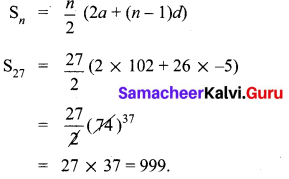(iii) 6 + 13 + 20 + … + 97
a = 6,d = 7, l = 97Ex 2.6 Class 10 Samacheer Question 2.
How many consecutive odd integers beginning with 5 will sum to 480?
5,7,9, 11, 13,…
Sn = 480
a = 5, d = 2, Sn = 480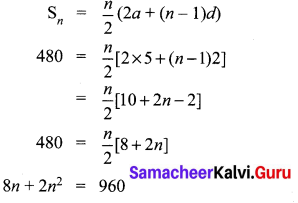2n2 + 8n – 960 = 0
⇒ n2 + 4n – 480 = 0
⇒ n2 + 24n – 20n – 480 = 0
⇒ n(n + 24) – 20(n + 24) = 0
⇒ (n – 20)(n + 24) = 0
⇒ n = 20,-24
No. of terms cannot be -ve.
∴ No. of consecutive odd integers beginning with 5 will sum to 480 is 20.

10th Maths Exercise 2.6 Solution Question 3.
Find the sum of first 28 terms of an A.P. whose nth term is 4n – 3.
Number of terns (n) = 28
tn = 4n – 3
t1 = 4(1) – 3 = 4 – 3 = 1
t2 = 4(2) – 3 = 8 – 3 = 5
t3 = 4(3) – 3 = 12 – 3 = 9
Here a = 1, d = 5 – 1 = 4
S28 = n2 [2a + (n – 1)d]
= 282 [2 + (27) (4)]
= 14 [2 + 108]
= 14 × 110
= 1540
Sum of 28 terms = 1540

10th Maths Exercise 2.6 Question 4.
The sum of first n terms of a certain series is given as 2n2 – 3n. Show that the series is an A.P.
Solution:
Given Sn = 2n2 – 3n
S1 = 2(1)2 – 3(1) = 2 – 3 = – 1
⇒ t1 = a = – 1
S2 = 2(22) – 3(2) = 8 – 6 = 2
t2 = S2 – S1 = 2 – (-1) = 3
∴ d = t2 – t1 = 3 – (-1) = 4
Consider a, a + d, a + 2d, ….….
-1, -1 + 4, -1 + 2(4), …..…
-1, 3, 7,….
Clearly this is an A.P with a = – 1, and d = 4.

Exercise 2.6 Class 10 Maths Question 5.
The 104th term and 4th term of an A.P are 125 and 0. Find the sum of first 35 terms.
Solution:
t104 = 125
t4 = 0
a + (n – 1)d = tn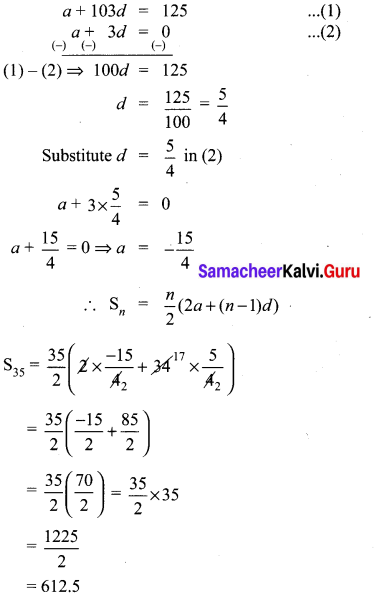10th Maths Exercise 2.6 In Tamil Question 6.
Find the sum of all odd positive integers less than 450.
Solution:
Sum of all odd positive integers less than 450 is given by
1 + 3 + 5 + … + 449
a = 1
d = 2
l = 449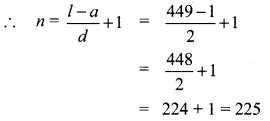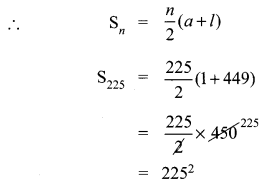= 50625
Another method:
Sum of all +ve odd integers = n2.
We can use the formula n2 = 2252
= 50625Ex 2.6 Class 10 Question 7.
Find the sum of all natural numbers between 602 and 902 which are not divisible by 4.
Natural numbers between 602 and 902
603,604, …, 901
a = 603, l = 901, d = 1,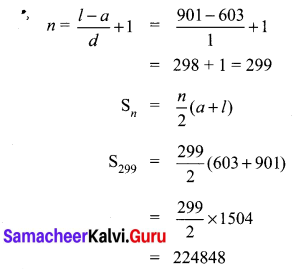Sum of all natural numbers between 602 and 902 which are not divisible by 4.
= Sum of all natural numbers between 602 and 902
= Sum of all natural numbers between 602 and 902 which are divisible by 4.
l = 902 – 2 = 900
To make 602 divisible by 4 we have to add 2 to 602.
∴ 602 + 2 = 604 which is divisible by 4.
To make 902 divisible by 4, subtract 2 from 902.
∴ 900 is the last number divisible by 4.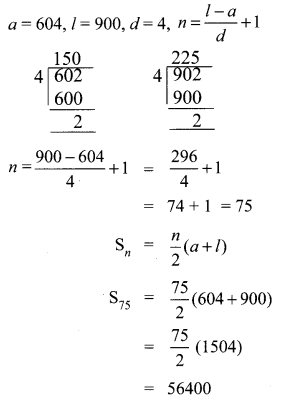Sum of all natural numbers between 602 and 902 which are not divisible 4.
= 224848 – 56400
= 168448

Exercise 2.6 Class 10 Maths Solution Question 8.
Raghu wish to buy a Laptop. He can buy it by paying ₹40,000 cash or by making 10 installments as ₹4800 in the first month, ₹4750 in the second month, ₹4700 in the third month and so on. If he pays the money in this fashion, Find
(i) Total amount paid in 10 installments.
(ii) How much extra amount that he pay in installments.
(i) Amount paid in 10 installments
4800 + 4750 + 4700 + ……………. 10
Here a = 4800; d = – 50 ; n = 10
Sn = n2 [2a +(n – 1)d]
S10 = 102 [2 × 4800 +9(-50)]
= 102 [9600 – 450]
= 5 
= 45750
Amount paid in 10 installments
= ₹45750
(ii) Extra amount paid = amount paid in 10
installment – cost of the laptop
= ₹45750 – 40,000
= ₹ 5750
(i) Amount paid in 10 installments = ₹ 45750
(ii) Difference in payment = ₹ 5750

Class 10 Maths Exercise 2.6 Solution Question 9.
A man repays a loan of ₹65,000 by paying ₹400 in the first month and then increasing the payment by ₹300 every month. How long will it take for him to clear the loan?
Solution:
Loan Amount = ₹ 65,000
Repayment through installments
400 + 700 + 1000 + 1300 + …
a = 400
d = 300
Sn = 65000
Sn = n2 (2a + (n – 1)d)
= 65000
n2(2 × 400 + (n – 1)300) = 65000
n(800 + 300n – 300) = 130000
n(500 + 300n) = 130000
500n + 300n2 = 130000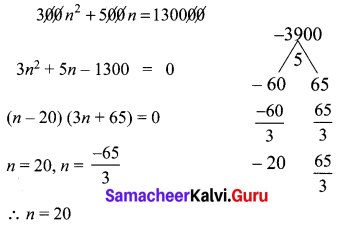Number of terms should be (+ve) and cannot be (-ve) or fractional number.
∴ He will take 20 months to clear the loans.

2.6 Maths Class 10 Question 10.
A brick staircase has a total of 30 steps. The bottom step requires 100 bricks. Each successive step requires two bricks less than the previous step.
(i) How many bricks are required for the top most step?
(ii) How many bricks are required to build the stair case?
Total number of steps = 30
∴ n = 30
Number of bricks for the bottom = 100
a = 100
2 bricks is less for each step

(i) Number of bricks required for the top most step
tn = a + (n – 1)d
t30 = 100 + 29 (-2)
= 100 – 58
= 42

(ii) Number of bricks required
Sn = n2 [2a + (n-1) d]
S30 = 302 [200 + 29 (-2)]
= 15[200 – 58]
= 2130
(i) Number of bricks required for the top most step = 42 bricks
(ii) Number of bricks required = 2130

Exercise 2.6 Class 10 Question 11.
If S1,S2,S3 , …. , Sm are the sums of n terms of m A.P.’s whose first terms are 1,2,3,…,m and whose common differences are 1, 3, 5,…, (2m – 1) respectively, then show that
S1 + S2 + S3 + …. + Sm = 12 mn(mn + 1).
Solution: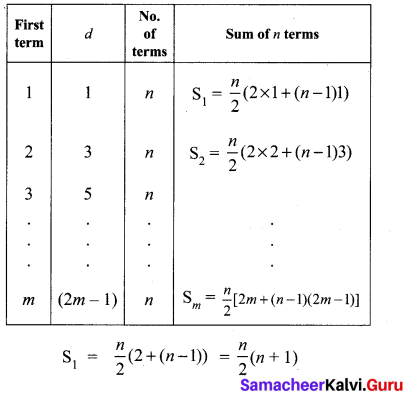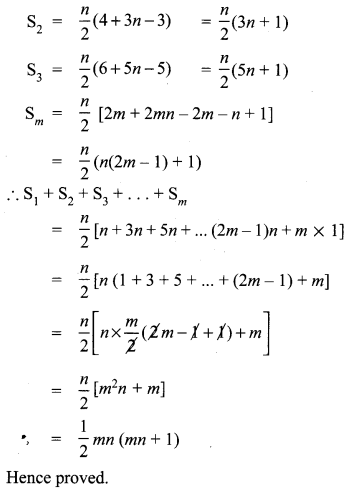Question 12.
Find the sum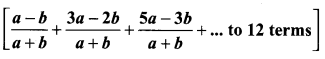Solution: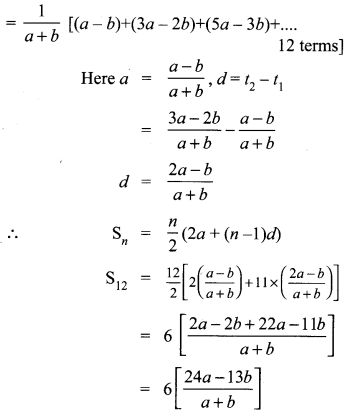## Tamilnadu Samacheer Kalvi 10th Maths Solutions Chapter 2 Numbers and Sequences Ex 2.7

10th Maths Exercise 2.7 Samacheer Kalvi Question 1.
Which of the following sequences are in G.P?
(i) 3, 9, 27, 81, ……..
(ii) 4, 44, 444, 4444, ………
(iii) 0.5, 0.05, 0.005, ……..
(iv) 13,16,112………
(v) 1, -5, 25, -125, …….
(vi) 120, 60, 30, 18, …….
(vii) 16, 4, 1, 14, ……
Solution:
(i) 3, 9, 27, 81
r = Common ratio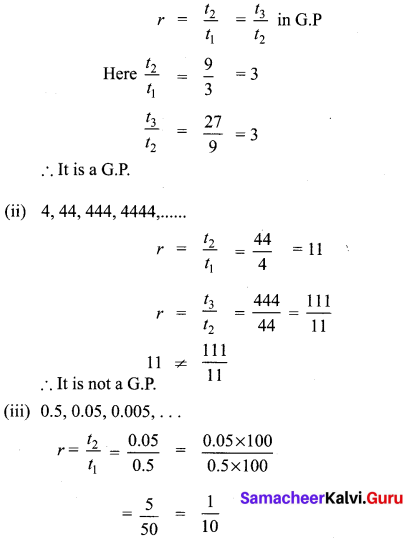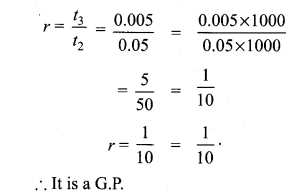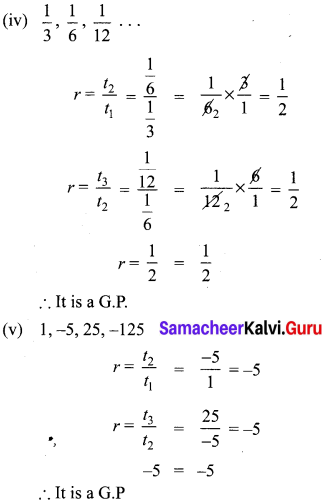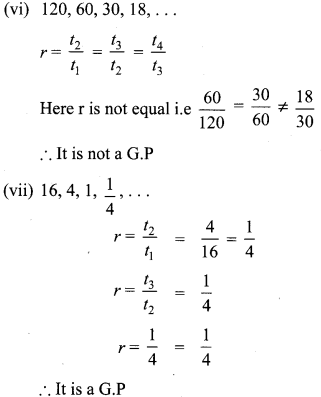10th Maths Exercise 2.7 Question 2.
Write the first three terms of the G.P. whose first term and the common ratio are given below.
(i) a = 6, r = 3
(ii) a = 2–√ , r = 2–√
(iii) a = 1000, r = 25
Solution:
(i) a = 6, r = 3
tn = arn-1
t1 = ar1-1 = ar0 = a = 6
t2 = ar2-1 = ar1 = 6 × 3 = 18
t3 = ar3-1 = ar2 = 6 × 32 = 54
∴ The 3 terms are 6, 18, 54, ….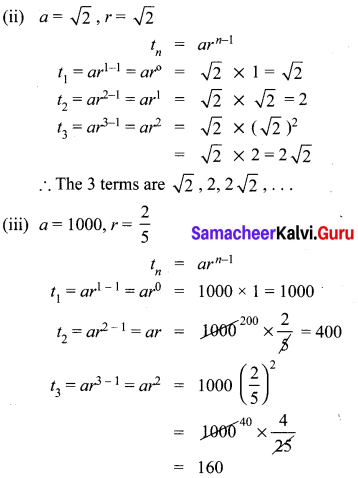The 3 terms are 1000, 400, 160, ……………

Ex 2.7 Class 10 Samacheer Question 3.
In a G.P. 729, 243, 81,… find t7.
Solution:
G.P = 729, 243, 81 ……
t7 = ?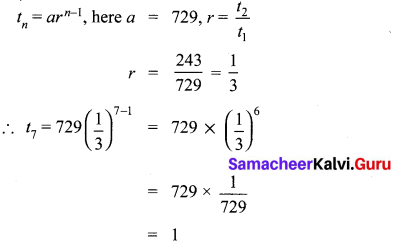Ex 2.7 Class 10 Question 4.
Find x so that x + 6, x + 12 and x + 15 are consecutive terms of a Geometric Progression.
t2t1 = x+12x+6,t3t2 = x+15x+12
Since it is a G.P.
x+12x+6 = x+15x+12
(x + 12)2 = (x + 6) (x + 15)
x2 + 24x + 144 = x2 + 21x + 90
3x = -54 ⇒ x = 543 = -18

Exercise 2.7 Class 10 Maths Question 5.
Find the number of terms in the following G.P.
(i),4, 8, 16, …, 8192
(ii) 13,19,127,……12187
Solution:
(i) 4, 8, 16, …… 8192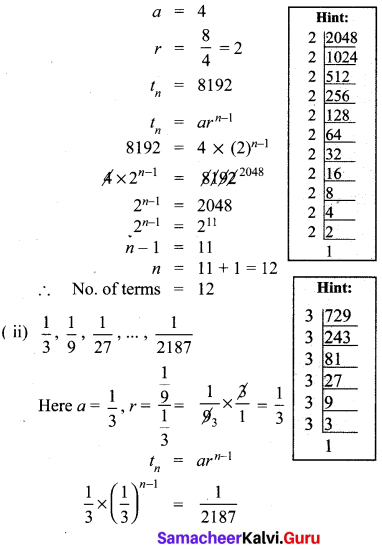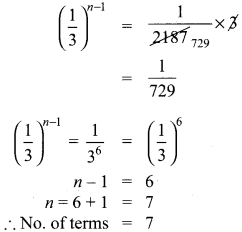10th Maths 2.7 Exercise Question 6.
In a G.P. the 9th term is 32805 and 6th term is 1215. Find the 12th term.
Solution:
In a G.P
tn = arn-1
t9 = 32805
t6 = 1215
t12 = ?
Let
t9 = ar8 = 32805 ………(1)
t6 = ar5 = 1215 ………. (2)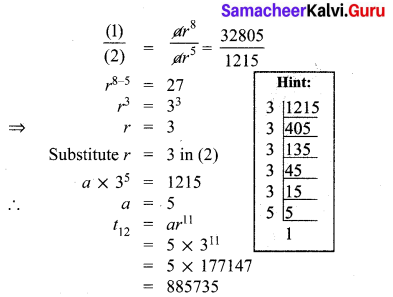10th Math Exercise 2.7 Solution Question 7.
Find the 10th term of a G.P. whose 8th term is 768 and the common ratio is 2.
Here r = 2, t8 = 768
t8 = 768 (tn = arn-1)
a. r8-1 = 768
ar7 = 768 …..(1)
10th term of a G.P. = a.r 10-1
= ar9
= (ar7) × (r2)
= 768 × 22 (from 1)
= 768 × 4 = 3072
∴ 10th term of a G.P. = 3072

Class 10 Maths Exercise 2.7 Solutions Question 8.
If a, b, c are in A.P. then show that 3a,3b,3c are in G.P.
Solution:
If a, b, c are in A.P
t2 – t1 = t3 – t2
b – a = c – b
2b = c + a
To prove that 3a, 3b, 3c are in G.P
⇒ 32b = 3c+a + a [Raising the power both sides]
⇒ 3b.3b = 3c.3a
3b3a=3c3b
t2t1=t3t1
⇒ Common ratio is same for 3a,3b,3c
⇒ 3a, 3b, 3c forms a G.P
∴ Hence it is proved .

Exercise 2.7 Class 10 Maths Solution Question 9.
In a G.P. the product of three consecutive terms is 27 and the sum of the product of two terms taken at a time is 572. Find the three terms.
Solution:
Let the three consecutive terms in a G.P are ar, a, ar.
Their Product = ar × a × ar = 27
a3 = 27 = 33
a = 3
Sum of the product of terms taken two at a time is 572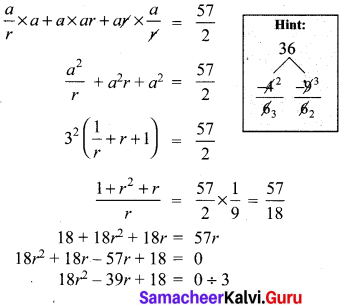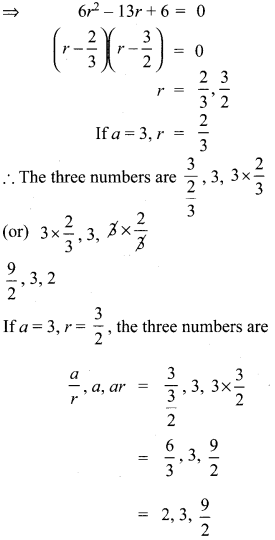2.7 Exercise Class 10 Question 10.
A man joined a company as Assistant Manager. The company gave him a starting salary of ₹60,000 and agreed to increase his salary 5% annually. What will be his salary after 5 years?
Solution:
Starting salary = ₹ 60,000
Increase per year = 5%
∴ At the end of 1 year the increase
= 60,0,00 × 5100
₹ 3000
∴ At the end of first year his salary
= ₹ 60,000 + 3000
I year salary = ₹ 63,000
II Year increase = 63000 × 5100
At the end of II year, salary
= 63000 + 3150
= ₹ 66150
III Year increase = 66150 × 5100
= 3307.50
At the end of III year, salary = 66150 + 3307.50
= ₹ 69457.50
IV year increase = 69457.50 × 5100
= ₹ 3472.87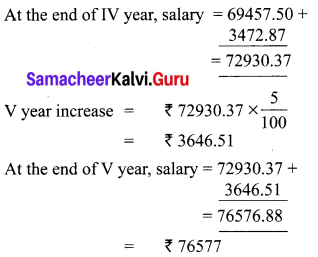Exercise 2.7 Class 10 Question 11.
Sivamani is attending an interview for a job and the company gave two offers to him. Offer A: ₹ 20,000 to start with followed by a guaranteed annual increase of 3% for the first 5 years.
Offer B: ₹ 22,000 to start with followed by a guaranteed annual increase of 3% for the first 5 years.
What is his salary in the 4th year with respect to the offers A and B?
Solution:
Offer A
Starting salary ₹ 20,000
Annual increase 6%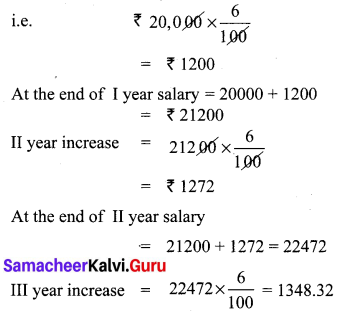At the end of
III year ,salary = 22472 + 1348 = 23820
∴ IV year salary = ₹ 23820
Offer B
Starting salary = ₹ 22,000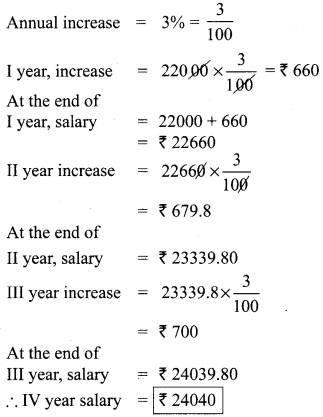Salary as per Option A = ₹ 23820
Salary as per Option B = ₹ 24040
∴ Option B is better.

10 Class Math Exercise 2.7 Solution Pdf Question 12.
If a, b, c are three consecutive terms of an A.P. and x, y, z are three consecutive terms of a G.P. then prove that xb-c × yc-a × za-b = 1.
Solution:
a, b, c are three consecutive terms of an AP.
∴ Let a, b, c be a, a + d, a + 2d respectively ………… (1)
x, y, z are three consecutive terms of a GP.
∴ Assume x, y, z as x, x.r, x.r2 respectively ……… (2)
PT : xb-c , yc-a , za-b = 1
Substituting (1) and (2) in LHS, we get
LHS = xa+d-a-2d × (xr)a+2d-a × (xr2)a-a-d
= (x)-d . (xr)2d (xr2)-d
= 1xd × x2d . r2d × 1xdr2d = 1 = RHS

## Tamilnadu Samacheer Kalvi 10th Maths Solutions Chapter 2 Numbers and Sequences Ex 2.8

10th Maths Exercise 2.8 Question 1.
Find the sum of first n terms of the G.P.
(i) 5, -3, 95, – 2725, ……….
(ii) 256, 64, 16, ……..
Solution:
(i) 5, -3, 95, 2725, ………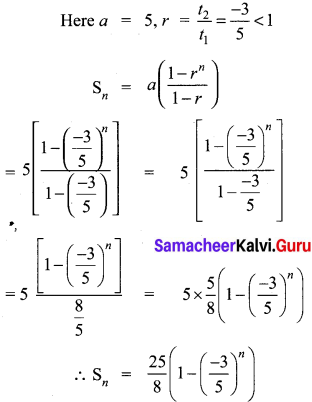(ii) 256, 64, 16,…….
a = 256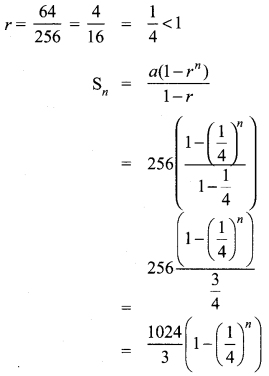Ex 2.8 Class 10 Samacheer Question 2.
Find the sum of first six terms of the G.P. 5, 15, 45, …
Solution:
G.P.= 5, 15, 45, 15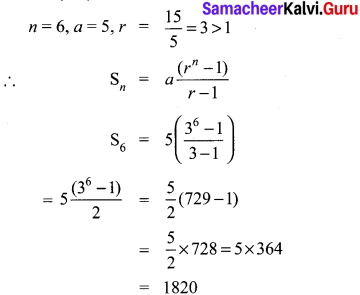Exercise 2.8 Class 10 Maths Solution Question 3.
Find the first term of the GP. whose common ratio 5 and whose sum to first 6 terms is 46872.
Solution:
Common ratio, r = 5
S6 = 46872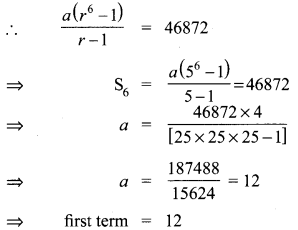10th Maths Exercise 2.8 Samacheer Kalvi Question 4.
Find the sum to infinity of (i) 9 + 3 + 1 + ….
(ii) 21 + 14 + 283 + ….
Solution:
(i) 9 + 3 + 1 + ….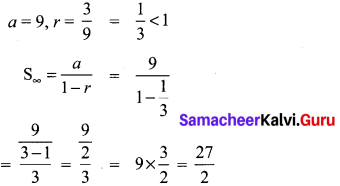(ii) 21 + 14 + 283 + ….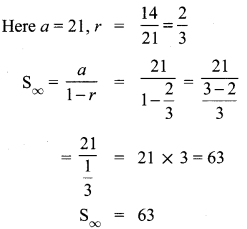Exercise 2.8 Class 10 Maths Question 5.
If the first term of an infinite G.P. is 8 and its sum to infinity is 323 then find the common ratio.
Solution:
a = 8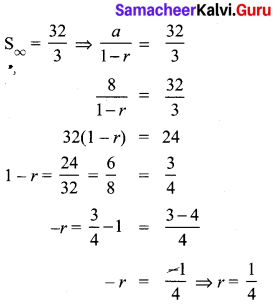2.8 Maths Class 10 Question 6.
Find the sum to n terms of the series
(i) 0.4 + 0.44 + 0.444 +…. to n terms
(ii) 3 + 33 + 333 + …. to n terms
Solution:
(i) 0.4 + 0.44 + 0.444 + … to n terms
= 4 (0.1 + 0.11 + 0.111 + … to n terms)
= 49 (0.9 + 0.99 + 0.999 + … to n terms)
= 49 (1 -0.1) + (1 – 0.01) +(1 – 0.001) + … n terms)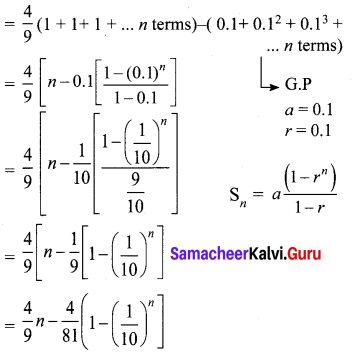(ii) 3 + 33 + 333 + … to n terms.
= 3 (1 + 11 + 111 + …….. to n terms)
= 39 (9 + 99 + 999 +… to n terms)
13 = [(10 – 1) + (100 – 1) + (1000 – 1) + … to n terms]
= 13 [(10 + 100 + 1000 + .. ) + (-1)n]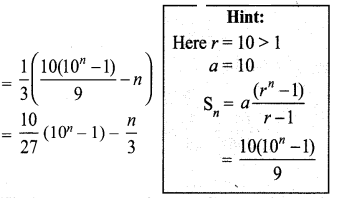2.8 Exercise Class 10 Question 7.
Find the sum of the Geometric series
3 + 6 + 12 + …… + 1536.
Solution:
3 + 6 + 12 + …… + 1536
Here a = 3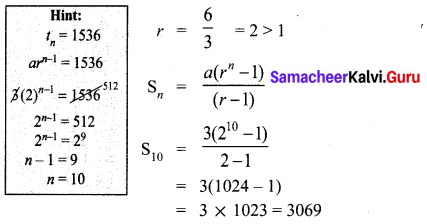Ex 2.8 Class 10 Question 8.
Kumar writes a letter to four of his friends. He asks each one of them to copy the letter and mail to four different persons with the instruction that they continue the process similarly. Assuming that the process is unaltered and it costs ₹2 to mail one letter, find the amount spent on postage when 8th set of letters is mailed.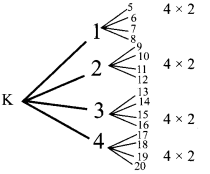Solution:
Kumar (1) 2 × 4
Cost of posting 1st set of Letters.
Cost of posting 2nd set of letters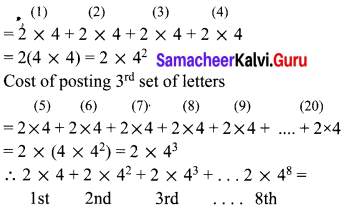Amount spent
[a + ar + ar2 +… arn-1]
= 4 [2 + 2 × 4 + 2 × 42 ÷ + 2 × 47]
= 4 [Sn] Here n = 8, r = 4
It is a G.P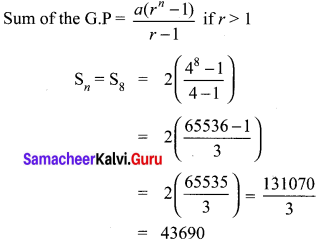∴ Cost of postage after posting 8th set of letters
= 4 × 43690 = ₹ 174760

Class 10 Maths Exercise 2.8 Solutions Question 9.
Find the rational form of the number 0.123¯¯¯¯¯¯¯¯
Solution:
Let x = 0.123123123 ………. ⇒ x = 0.123¯¯¯¯¯¯¯¯ …..(1)
Multiplying 1000 on both rides
1000 x = 123.123123… ⇒ 1000x = 123.123¯¯¯¯¯¯¯¯ …..(2)
(2) – (1) = 1000x – x 123.123¯¯¯¯¯¯¯¯ – 0.123¯¯¯¯¯¯¯¯.
⇒ 999 x = 123
⇒ x = 123999
⇒ x = 41333 Rational number

Exercise 2.8 Class 10 Question 10.
If Sn = (x + y)+(x2 + xy + y2) + (x3 + x2y + xy2 + y3) + …n terms then prove that (x – y)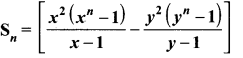Solution:
Sn = (x + y) + (x2 + xy + y2) + (x3 + x2y + xy2 + y3) + …n terms
⇒ x. Sn = (x + y)x + (x2 + xy + y2)x + (x3 + x2y + xy2 + y3) x + ….
⇒ x. Sn = x2 +xy + x3 + x2y + y2x + x4 + x3y
+ x2 y2 + y3 x +…… ….(1)
Multiplying ‘y’ on both sides,
= y.Sn = (x + y)y + (x2 + xy + y2) y+
(x3 + x2y + xy2 + y3) y + ….
= y.Sn = xy + y2 + x2y + xy2 + y3 + x3y
+ x3y + x2y2 + xy3 + y4 + ……….
(1) – (2) ⇒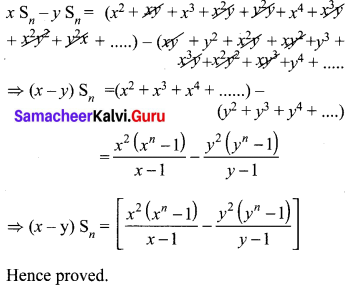## Tamilnadu Samacheer Kalvi 10th Maths Solutions Chapter 2 Numbers and Sequences Ex 2.9

10th Maths Exercise 2.9 Samacheer Kalvi Question 1.
Find the sum of the following series
(i) 1 + 2 + 3 + … + 60
(ii) 3 + 6 + 9 + … + 96
(iii) 51 + 52 + 53 + … + 92
(iv) 1 + 4 + 9 + 16 + … + 225
(v) 62 + 72 + 82 + … + 212
(vi) 103 + 113 + 123 + … + 203
(vii) 1 + 3 + 5 + … + 71
Solution:
(i) 1 + 2 + 3 + ……… + 60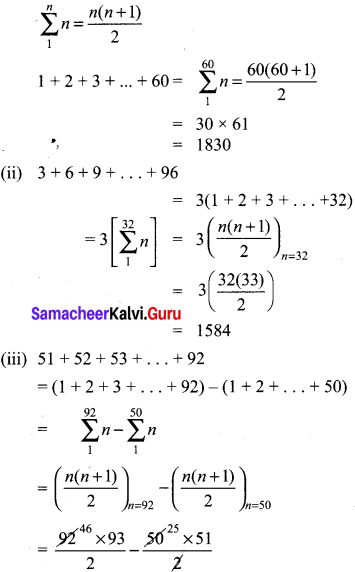= 4278 – 1275 = 3003
(iv) 1 + 4 + 9 + 16 + … + 225
= 12 + 22 + 32 + 42 + ……… + 152
n1n2=n(n+1)(2n+1)6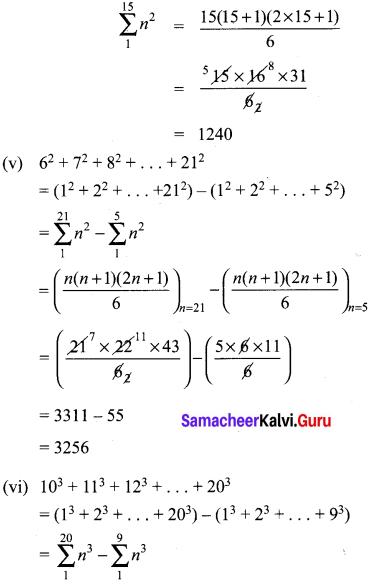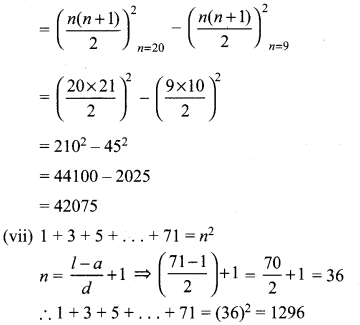Ex 2.9 Class 10 Samacheer Question 2.
If 1 + 2 + 3 + … + k = 325, then find 13 + 23 + 33 + …………. + K3.
Solution:
1 + 2 + 3 + … + K = 325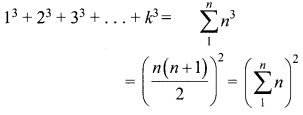If 1 + 2 + 3 … + k = 325
13 + 23 + 33 + … + K3 = (325)2 = 105625

10th Maths Exercise 2.9 Answers Question 3.
If 13 + 23 + 33 + … + K3 = 44100 then find 1 + 2 + 3 + … + k.
Solution:
If 13 + 23 + 33 + … + K3 = 44100
1 + 2 + 3 + … + K = 44100−−−−−√
= 210

Exercise 2.9 Class 10 Maths Question 4.
How many terms of the series 13 + 23 + 33 + … should be taken to get the sum 14400?
Solution:
13 + 23 + 33 + ……… + n3 = 14400
(n(n+1)2)2 = 14400 = (120)2
n(n+1)2 = 14400−−−−−√ = 120
n(n + 1) = 240
Method 1:
n2 + n – 240 = 0
n2 + 16n – 15n – 240 = 0
n(n + 16) – 15(n + 16) = 0
(n + 16)(n – 15) = 0
n = -16, 15
∴ 15 terms to be taken to get the sum 14400.
Method 2:
n2 + n – 240 = 0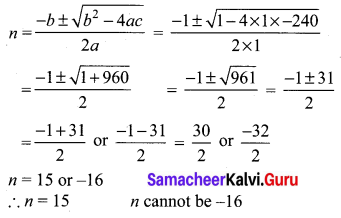Exercise 2.9 Class 10 Maths Samacheer Question 5.
The sum of the squares of the first n natural numbers is 285, while the sum of their cubes is 2025. Find the value of n.
Solution:
12 + 22 + 32 + …… + n2 = 285
13 + 23 + 33 + …… + n3 = 2025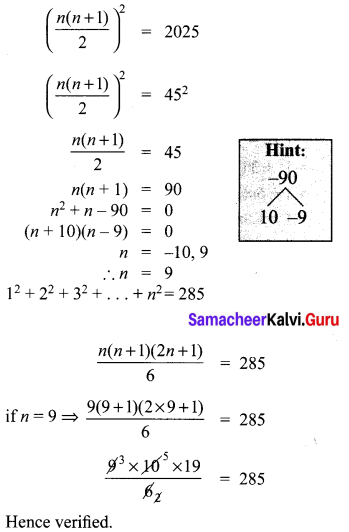10th Maths Exercise 2.9 Question 6.
Rekha has 15 square colour papers of sizes 10 cm, 11 cm, 12 cm,…, 24 cm. How much area can be decorated with these colour papers?
Solution:
102 + 112 + 122 + … + 242
= (12 + 22 + … + 242) – (12 + 22 + … + 92)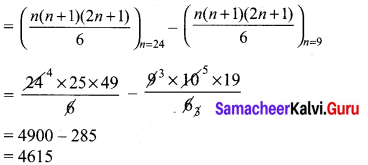∴ Rekha has 4615 cm2 colour papers. She can decorate 4615 cm2 area with these colour papers.

Ex 2.9 Class 10 Question 7.
Find the sum of the series (23 – 1) + (43 – 33) + (63 – 153) +… to (i) n terms (ii) 8 terms.
Solution:
(23 – 1) + (43 – 33) + (63 – 153) + ……… n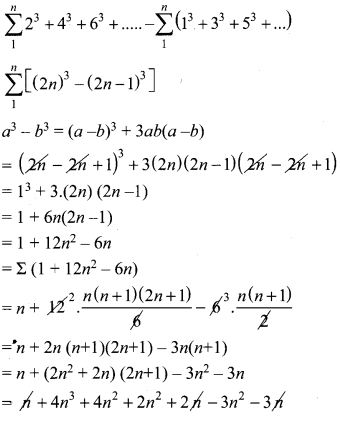= 4n3 + 3n2 = sum of ‘n’ terms.
When n = 8
Sum = 4 × 83 + 3 × 82
= 2048 + 192 = 2240

## Tamilnadu Samacheer Kalvi 10th Maths Solutions Chapter 2 Numbers and Sequences Ex 2.10

Multiple choice questions
Question 1.
Euclid’s division lemma states that for positive integers a and b, there exist unique integers q and r such that a = bq + r, where r must satisfy.
(1) 1 < r < b
(2) 0 < r < b
(3) 0 < r < b
(4) 0 < r < b
(3) 0 < r < b

Question 2.
Using Euclid’s division lemma, if the cube of any positive integer is divided by 9 then the possible remainders are
(1) 0, 1, 8
(2) 1, 4, 8
(3) 0, 1, 3
(4) 1, 3, 5
(1) 0,1,8
Hint:
Cube of any +ve integers 13, 23, 33, 43,. . .
1, 8, 27, 64, 125, 216 …
Remainders when 27, 64, 125 are divided by 9.

Question 3.
If the H.C.F of 65 and 117 is expressible in the form of 65m -117 , then the value of m is ………………….
(1) 4
(2) 2
(3) 1
(4) 3
(2) 2
Hint:
117 = 3 × 3 × 13
65 = 5 × 13
H.C.F = 13
65m – 117 = 13 ⇒ 65m = 117 + 13 = 130
m = 13065 = 2
The value of m = 2

Question 4.
The sum of the exponents of the prime factors in the prime factorization of 1729 is
(1) 1
(2) 2
(3) 3
(4) 4
(3) 3
Hint:
1729 = 71 × 131 × 191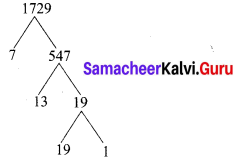Question 5.
The least number that is divisible by all the numbers from 1 to 10 (both inclusive) is
(1) 2025
(2) 5220
(3) 5025
(4) 2520
(4) 2520
Hint: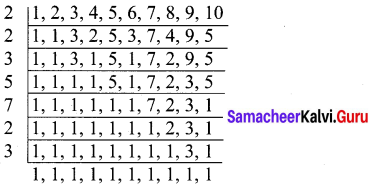∴ L.C.M. of 1,2,3,4,…,10 is 2 × 2 × 3 × 5 × 7 × 2 × 3 = 2520

Question 6.
74k ≡ …………………. (mod 100)
(1) 1
(2) 2
(3) 3
(4) 4
(1) 1
Hint:
74k ≡ . . . . . (mod 100)
74k = (74)k ≡ ……….. (mod 100) (74 – 2401)
The value is 1.

Question 7.
Given F1 = 1, F2 = 3 and Fn = Fn-1 + Fn-2 then
(1) 3
(2) 5
(3) 8
(4) 11
(4) 11
F1 = 1, F2 = 3
Fn = Fn-1 + Fn-2
F5 = F5-1 + F5-2 = F4 + F3
= F3 + F2 + F2 + F1
= F2 + F1 + F2 + F2 + F1
= 3 + 1 + 3 + 3 + 1 = 11

Question 8.
The first term of an arithmetic progression is unity and the common difference is 4. Which of the following will be a term of this A.P …………..
(1) 4551
(2) 10091
(3) 7881
(4) 13531
(3) 7881
Hint:
Here a = 1, d = 4
tn = a + (n – 1) d = 1 + (n – 1) 4
= 1 + 4n – 4
= 4n – 3
4554
(i) 4n – 3 = 4551 ⇒ 4n = 4551 + 3 ⇒ n = 45544 = 1138.5.
It is not a term of A.P.

(ii) 4n – 3 = 10091 ⇒ 4n = 10091 + 3 = 10094
n = 100944 = 2523.5 it is a term of A.P.

(iii) 4n – 3 = 7881 ⇒ 4n = 7881 + 3
n = 78844 = 1971.
∴ 7881 is a term of the A.P.

Question 9.
If 6 times of 6th term of an A.P is equal to 7 times the 7th term, then the 13th term of the A.P. is
(1) 0
(2) 6
(3) 7
(4) 13
(1) 0
Hint:
6t6 = 7t7
6(a + 5d) = 7(a + 6d)
6a + 30d = 7a + 42d
7a + 42d – 6a – 30d = 0
a + 12d = 0 = t13

Question 10.
An A.P consists of 31 terms. If its 16th term is m, then the sum of all the terms of this A.P. is …………..
(1) 16m
(2) 62m
(3) 31m
(4) 312m
(3) 31m
Hint:
M = 31
t16 = m ⇒ a + 15d = m
Sn = n2[2a + (n – 1)d]
Sn = 312[2a + 30d]= 312 × 2[a + 15d]
= 31 (m) = 31m

Question 11.
In an A.P., the first term is 1 and the common difference is 4. How many terms of the A.P must be taken for their sum to be equal to 120?
(1) 6
(2) 7
(3) 8
(4) 9
(3) 8
Hint: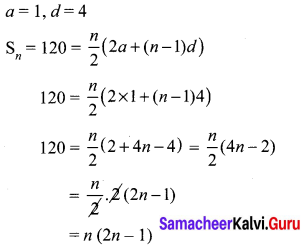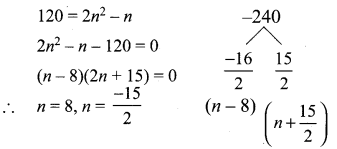Question 12.
If A = 265 and B = 264 + 263 + 262 + +20 which of the following is true?
(1) B is 264 more than A
(2) A and B are equal
(3) B is larger than A by 1
(4) A is larger than B by 1
(4) A is larger than B by 1
Hint:
A = 265
B = 264 + 263 + 262 + … + 20
B = 20 + 21 + 22 + … + 264
G.P = 1 + 21 + 22 + … + 264 it is a G.P
Here a = 1, r = 2, n = 65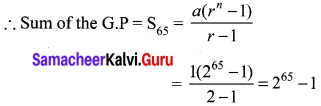A = 265, B = 265 – 1
∴ B is smaller.
A is larger than B by 1.

Question 13.
The next term of the sequence 316,18,112,118, ….
(1) 124
(2) 127
(3) 23
(4) 181
(2) 127
Hint: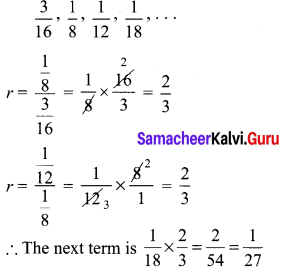Question 14.
If the sequence t1, t2, t3, …………are in A.P. then the sequence t6, t12, t18, …… is ………….
(1) a Geometric progression
(2) an Arithmetic progression
(3) neither an Arithmetic progression nor a Geometric progression
(4) a constant sequence
(2) an Arithmetic progression
Hint: t1, t2, t3 …. are in A.P
t6, t12, t18 …… is also an A.P. (6, 12, 18 …….. is an A.P.)

Question 15.
The value of (13 + 23 + 33 + … + 153) – (1 + 2 + 3 + … + 15) is
(1) 14400
(2) 14200
(3) 14280
(4) 14520
(3) 14280
Hint:
(15×162)215×162 = (120)2 – 120 = 14280

## Tamilnadu Samacheer Kalvi 10th Maths Solutions Chapter 2 Numbers and Sequences Unit Exercise 2

Question 1.
Prove that n2 – n divisible by 2 for every positive integer n.
To prove n2 – n divisible by 2 for every positive integer n.
We know that any positive integer is of the form 2q or 2q + 1, for some integer q.
So, following cases arise:
Case I. When n = 2q.
In this case, we have
n2 – n = (2q)2 – 2q = 4q2 – 2q = 2q(2q – 1)
⇒ n2 – n = 2r where r = q(2q – 1)
⇒ n2 – n is divisible by 2.

Case II. When n = 2q + 1.
In this case, we have
n2 – n = (2q + 1)2 – (2q + 1)
= (2q + 1)(2q + 1 – 1) = (2q + 1)2q
⇒ n2 – n = 2r where r = q (2q + 1)
⇒ n2 – n is divisible by 2.
Hence n2 – n is divisible by 2 for every positive integer n.

Question 2.
A milk man has 175 litres of cow’s milk and 105 litres of buffalow’s milk. He wishes to sell the milk by filling the two types of milk in cans of equal capacity. Calculate the following (i) Capacity of a can
(ii) Number of cans of cow’s milk
(iii) Number of cans of buffalow’s milk.
Cow’s milk = 175 litres
Buffalow’s milk = 105 litres
Find the H.C.F. of 175 and 105 using Euclid’s division method of factorisation method.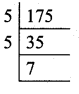175 = 5 × 5 × 7
105 = 3 × 5 × 7
H.C.F. of 175 and 105 = 5 × 7 = 35
(i) The capacity of the milk can’s is 35 litres

(ii) Cows milk = 175 litres
Number of cans = 17535 = 5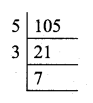(iii) Buffalow’s milk = 105 litres
Number of cans = 10535 = 3
(i) Capacity of one can = 35 litres
(ii) Number of can’s for cow’s milk= 5 litres
(iii) Number of can’s for Buffalow’s milk = 3 litres

Question 3.
When the positive integers a, b and c are divided by 13 the respective remainders are 9, 7 and 10. Find the remainder when a + 2b + 3c is divided by 13.
Let the positive integers be a, b, and c.
a = 13q + 9
b = 13q + 7
c = 13q + 10
a + 2b + 3c = 13 q + 9 + 2(13q + 7) + 3(13q + 10)
= 13q + 9 + 269 + 14 + 39q + 30
= 78q + 53 = (13 × 6)q + 53
The remainder is 53.
But 53 = 13 × 4 + 1
∴ The remainder is 1

Question 4.
Show that 107 is of the form 4q + 3 for any integer q.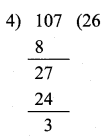107 = 4 x 26 + 3
This is in the form of a = bq + r
Hence it is proved.

Question 5.
If (m + 1)th term of an A.P. is twice the (n + 1)th term, then prove that (3m + 1)th term is twice the (m + n + 1)th term.
Solution:
tn = a + (n – 1)d
tm+1 = a + (m + 1 – 1)d
= a + md
tn+1 = a + (n + 1 – 1)d
= a + nd
2(tn+1) = 2(a + nd)
tm+1 = 2tn+1 …………… (1)
⇒ a + md = 2(a + nd)
2a + 2nd – a – md = 0
a + (2n – m)d = 0
t(3m+1) = a + (3m + 1 – 1)d
= a + 3md
t(m+n+1) = a + (m + n + 1 – 1)d
= a + (m + n)d
2(t(m+n+1)) = 2(a + (m + n)d)
= 2a + 2md + 2nd
t(3m+1) = 2t(m+n+1) ………….. (2)
a + 3md = 2a + 2md + 2nd
2a + 2md + 2nd – a – 3md = 0
a – md + 2nd = 0
a + (2n – m)d = 0
∴ It is proved that t(3m+1) = 2t(m+n+1)

Question 6.
Find the 12th term from the last term of the A.P -2, -4, -6, … -100.
Solution: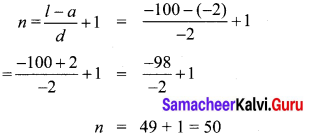12th term from the last = 39th term from the beginning
∴ t39 = a + 38d
= -2 + 38(-2)
= – 2 – 76
= – 78

Question 7.
Two A.P.’s have the same common difference. The first term of one A.P. is 2 and that of the other is 7. Show that the difference between their 10th terms is the same as the difference between their 21st terms, which is the same as the difference between any two corresponding terms.
Let the common difference for the 2 A.P be 'd'
For the first A.P
a = 2, d = d, n = 10
tn = a + (n – 1) d
t10 = 2 + 9 d ….(1)
For the 2nd A.P
a = 7, d = d n = 10
t10 = 7 + (9)d
= 7 + 9d ….(2)
Difference between their 10th term ⇒ (1) – (2)
= 2 + 9d – (7 + 9d)
= 2 + 9d – 7 – 9d
= -5
For first A.P when n = 21, a = 2, d = d
t21 = 2 + 20d …….(3)
For second A.P when n = 21, a = 7, d = d
t21 = 7 + 20d …….(4)
Difference between the 21st term ⇒ (3) – (4)
= 2 + 20d – (7 + 20d)
= 2 + 20d – 7 – 20d
= -5
Difference between their 10th term and 21st term = -5
Hence it is proved.

Question 8.
A man saved ₹16500 in ten years. In each year after the first he saved ₹100 more than he did in the preceding year. How much did he save in the first year?
Solution:
S10 = ₹16500
a, a + d, a + 2d…
d = 100
n = 10
Sn = n2(2a+(n-1)d)
S10 = 16500
S10 = 102(2×a+9×100)
16500 = 5(2a+900)
16500 = 10a + 4500
10a = 16500 – 4500
10a = 12000
a = 1200010 = ₹1200
∴ He saved ₹1200 in the first year

Question 9.
Find the G.P. in which the 2nd term is 6–√ and the 6th term is 96–√.
Solution: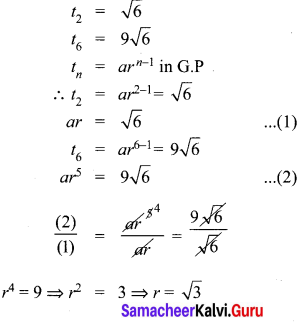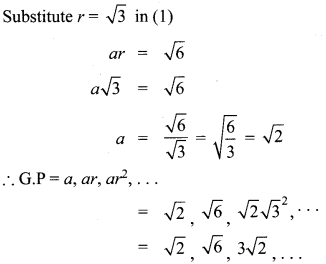Question 10.
The value of a motorcycle depreciates at the rate of 15% per year. What will be the value of the motor cycle 3 year hence, which is now purchased for ₹45,000?
Value of the motor cycyle = ₹ 45000
a = 45000
Depreciation = 15% of the cost value
= 15100 × 45000
= 15 × 450
= 6750
d = – 6750 (decrease it is depreciation
Value of the motor cycle lightning of the 2nd year = 45000 – 6750
= ₹ 38250
Depreciation for the 2nd year = 15100 × 38250
= ₹ 57370.50

## Tamilnadu Samacheer Kalvi 10th Maths Solutions Chapter 2 Numbers and Sequences Additional Questions

Question 1.
Use Euclid’s algorithm to find the HCF of 4052 and 12756.
Solution:
Since 12576 > 4052 we apply the division lemma to 12576 and 4052, to get HCF
12576 = 4052 × 3 + 420.
Since the remainder 420 ≠ 0, we apply the division lemma to 4052
4052 = 420 × 9 + 272.
We consider the new divisor 420 and the new remainder 272 and apply the division lemma to get
420 = 272 × 1 + 148, 148 ≠ 0.
∴ Again by division lemma
272 = 148 × 1 + 124, here 124 ≠ 0.
∴ Again by division lemma
148 = 124 × 1 + 24, Here 24 ≠ 0.
∴ Again by division lemma
124 = 24 × 5 + 4, Here 4 ≠ 0.
∴ Again by division lemma
24 = 4 × 6 + 0.
The remainder has now become zero. So our procedure stops. Since the divisor at this stage is 4.
∴ The HCF of 12576 and 4052 is 4.

Question 2.
If the HCF of 65 and 117 is in the form (65m – 117) then find the value of m.
By Euclid’s algorithm 117 > 65
117 = 65 × 1 + 52
52 = 13 × 4 × 0
65 = 52 × 1 + 13
H.C.F. of 65 and 117 is 13
65m – 117 = 13
65 m = 130
m = 13065 = 2
The value of m = 2

Question 3.
Find the LCM and HCF of 6 and 20 by the prime factorisation method.
Solution:
We have 6 = 21 × 31 and
20 = 2 × 2 × 5 = 22 × 51
You can find HCF (6, 20) = 2 and LCM (6, 20) = 2 × 2 × 3 × 5 = 60. As done in your earlier classes. Note that HCF (6, 20) = 21 = product of the smallest power of each common prime factor in the numbers.
LCM (6, 20) = 22 × 31 × 51 = 60.
= Product of the greatest power of each prime factor, involved in the numbers.

Question 4.
Prove that 3–√ is irrational.
Let us assume the opposite, (1) 3–√ is irrational.
Hence 3–√ = pq
Where p and q(q ≠ 0) are co-prime (no common factor other than 1)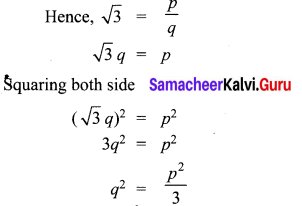Hence, 3 divides p2
So 3 divides p also …………….. (1)
Hence we can say
p3 = c where c is some integer
p = 3c
Now we know that
3q2 = p2
Putting = 3c
3q2 = (3c)2
3q2 = 9c2
q2 = 13 × 9c2
q2 = 3c2
q23 = C2
Hence 3 divides q2
So, 3 divides q also ……………. (2)
By (1) and (2) 3 divides both p and q

Question 5.
Which of the following list of numbers form an AP? If they form an AP, write the next two terms:
(i) 4, 10, 16, 22, …
(ii) 1, -1,-3, -5,…
(iii) -2, 2, -2, 2, -2, …
(iv) 1, 1, 1, 2, 2, 2, 3, 3, 3,…
Solution:
(i) 4, 10, 16, 22, …….
We have a2 – a1 = 10 – 4 = 6
a3 – a2 = 16 – 10 = 6
a4 – a3 = 22 – 16 = 6
∴ It is an A.P. with common difference 6.
∴ The next two terms are, 28, 34

(ii) 1, -1, -3, -5
t2 – t1 = -1 – 1 = -2
t3 – t2 = -3 – (-1) = -2
t4 – t3 = -5 – (-3) = -2
The given list of numbers form an A.P with the common difference -2.
The next two terms are (-5 + (-2)) = -7, -7 + (-2) = -9.

(iii) -2, 2,-2, 2,-2
t2 – t1 = 2-(-2) = 4
t3 – t2 = -2 -2 = -4
t4 – t3 = 2 – (-2) = 4
It is not an A.P.

(iv) 1, 1, 1, 2, 2, 2, 3, 3, 3
t2 – t1 = 1 – 1 = 0
t3 – t2 = 1 – 1 = 0
t4 – t3 = 2 – 1 = 1
Here t2 – t1 ≠ t3 – t2
∴ It is not an A.P.

Question 6.
Find n so that the nth terms of the following two A.P.’s are the same.
1, 7,13,19,… and 100, 95,90,…
The given A.P. is 1, 7, 13, 19,….
a = 1, d = 7 – 1 = 6
tn1 = a + (n – 1)d
tn1 = 1 + (n – 1) 6
= 1 + 6n – 6 = 6n – 5 … (1)
The given A.P. is 100, 95, 90,….
a = 100, d = 95 – 100 = – 5
tn2 = 100 + (n – 1) (-5)
= 100 – 5n + 5
= 105 – 5n …..(2)
Given that, tn1 = tn2
6n – 5 = 105 – 5n
6n + 5n = 105 + 5
11 n = 110
n = 10
∴ 10th term are same for both the A.P’s.

Question 7.
In a flower bed, there are 23 rose plants in the first row, 21 in the second, 19 is the third, and so on. There are 5 rose plants in the last row. How many rows are there in the flower bed?
The number of rose plants in the 1st, 2nd, 3rd,… rows are
23, 21, 19,………….. 5
It forms an A.P.
Let the number of rows in the flower bed be n.
Then a = 23, d = 21 – 23 = -2, l = 5.
As, an = a + (n – 1)d i.e. tn = a + (n – 1)d
We have 5 = 23 + (n – 1)(-2)
i.e. -18 = (n – 1)(-2)
n = 10
∴ There are 10 rows in the flower bed.

Question 8.
Find the sum of the first 30 terms of an A.P. whose nth term is 3 + 2n.
Given,
tn = 3 + 2n
t1 = 3 + 2 (1) = 3 + 2 = 5
t2 = 3 + 2 (2) = 3 + 4 = 7
t3 = 3 + 2 (3) = 3 + 6 = 9
Here a = 5,d = 7 – 5 = 2, n = 30
Sn = n2 [2a + (n – 1)d]
S30 = 302 [10 + 29(2)]
= 15 [10 + 58] = 15 × 68 = 1020
∴ Sum of first 30 terms = 1020

Question 9.
How many terms of the AP: 24, 21, 18, . must be taken so that their sum is 78?
Solution:
Here a = 24, d = 21 – 24 = -3, Sn = 78. We need to find n.
We know that,
Sn = n2 (2a + (n – 1)d)
78 = n2 (48 + 13(-3))
78 = n2 (51 – 3n)
or 3n2 – 51n + 156 = 0
n2 – 17n + 52 = 0
(n – 4) (n – 13) = 0
n = 4 or 13
The number of terms are 4 or 13.

Question 10.
The sum of first n terms of a certain series is given as 3n2 – 2n. Show that the series is an arithmetic series.
Solution:
Given, Sn = 3n2 – 2n
S1 = 3 (1)2 – 2(1)
= 3 – 2 = 1
ie; t1 = 1 (∴ S1 = t1)
S2 = 3(2)2 – 2(2) = 12 – 4 = 8
ie; t1 + t2 = 8 (∴ S2 = t1 + t2)
∴ t2 = 8 – 1 = 7
S3 = 3(3)2 – 2(3) = 27 – 6 = 21
t1 + t2 + t3 = 21 (∴ S3 = t1 + t2 + t3)
8 + t3 = 21 (Substitute t1 + t2 = 8)
t3 = 21 – 8 ⇒ t3 = 13
∴ The series is 1,7,13, …………. and this series is an A.P. with common difference 6.

## How to Prepare using Samacheer Kalvi 10th Maths Chapter 2 Numbers and Sequences Notes PDF?

Students must prepare for the upcoming exams from Samacheer Kalvi 10th Maths Chapter 2 Numbers and Sequences Notes PDF by following certain essential steps which are provided below.

• Use Samacheer Kalvi 10th Maths Chapter 2 Numbers and Sequences notes by paying attention to facts and ideas.
• Pay attention to the important topics
• Refer TN Board books as well as the books recommended.
• Correctly follow the notes to reduce the number of questions being answered in the exam incorrectly
• Highlight and explain the concepts in details.

## Frequently Asked Questions on Samacheer Kalvi 10th Maths Chapter 2 Numbers and Sequences Notes

#### How to use Samacheer Kalvi 10th Maths Chapter 2 Numbers and Sequences Notes for preparation??

Read TN Board thoroughly, make separate notes for points you forget, formulae, reactions, diagrams. Highlight important points in the book itself and make use of the space provided in the margin to jot down other important points on the same topic from different sources.

#### How to make notes for Samacheer Kalvi 10th Maths Chapter 2 Numbers and Sequences exam?

Read from hand-made notes prepared after understanding concepts, refrain from replicating from the textbook. Use highlighters for important points. Revise from these notes regularly and formulate your own tricks, shortcuts and mnemonics, mappings etc.
Share: